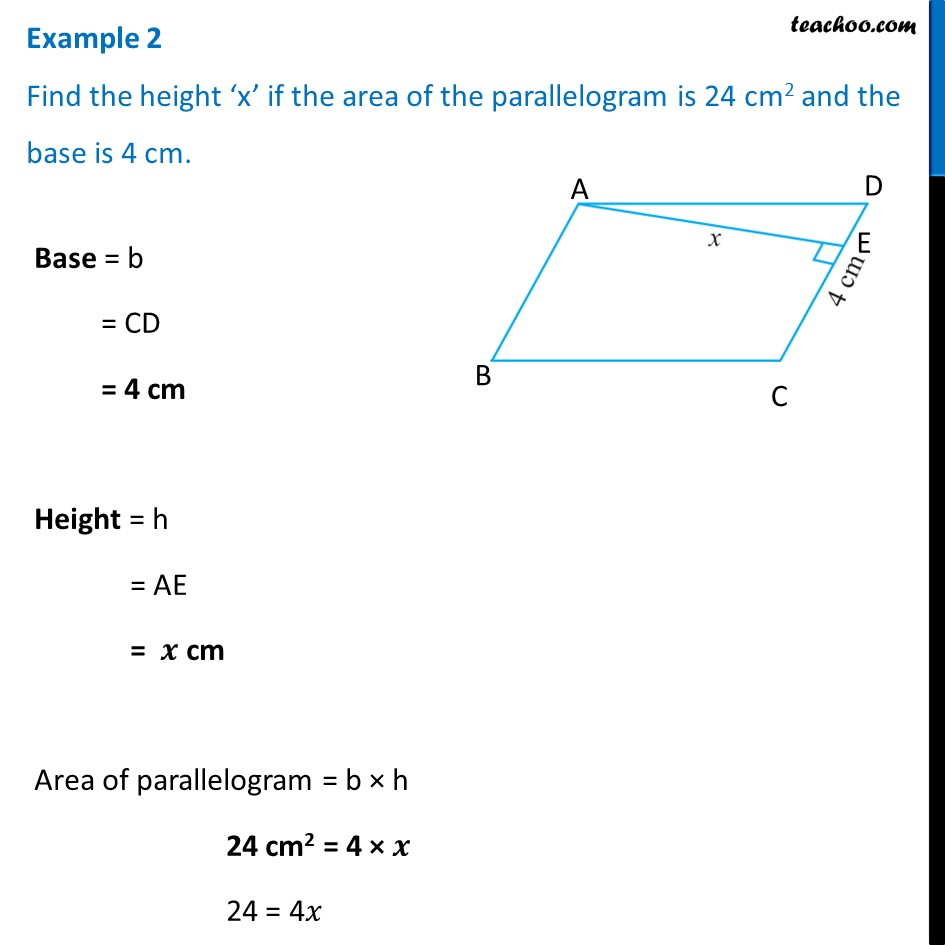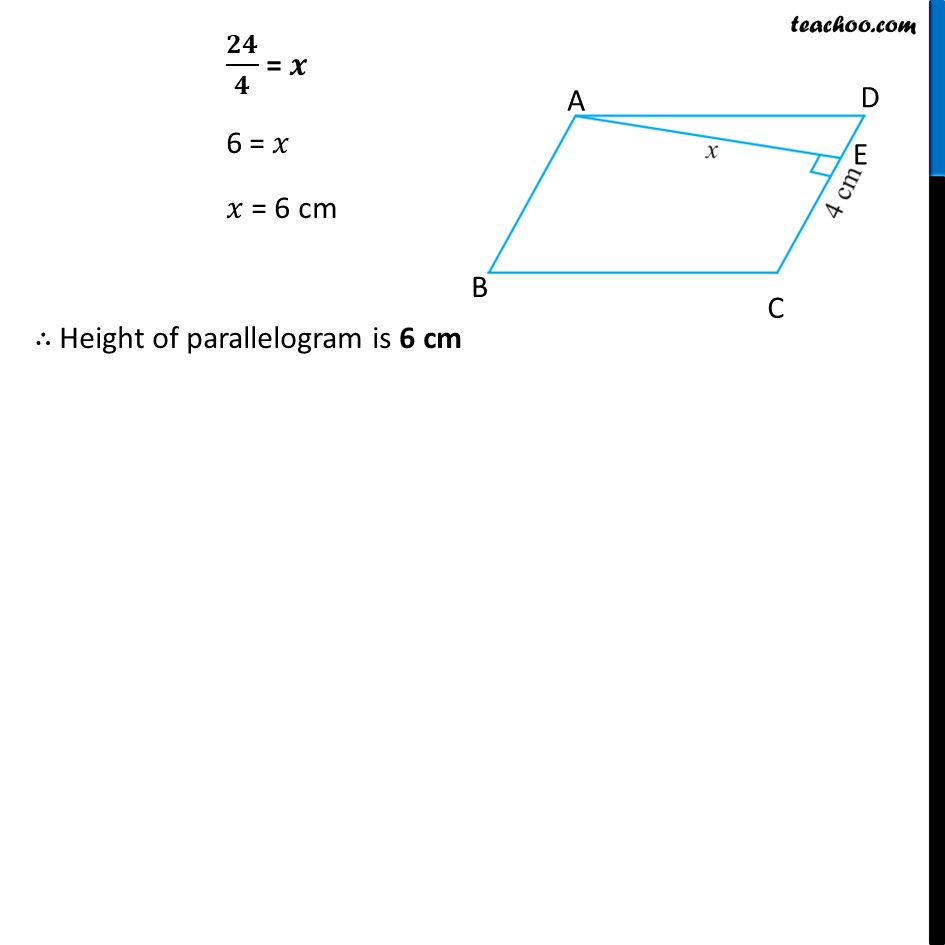Examples

Chapter 9 Class 7 Perimeter and Area
Serial order wiseLearn in your speed, with individual attention - Teachoo Maths 1-on-1 Class

### Transcript

Example 2 Find the height ‘x’ if the area of the parallelogram is 24 cm2 and the base is 4 cm.Base = b = CD = 4 cm Height = h = AE = 𝒙 cm Area of parallelogram = b × h 24 cm2 = 4 × 𝒙 24 = 4𝑥 𝟐𝟒/𝟒 = 𝒙 6 = 𝑥 𝑥 = 6 cm ∴ Height of parallelogram is 6 cm